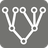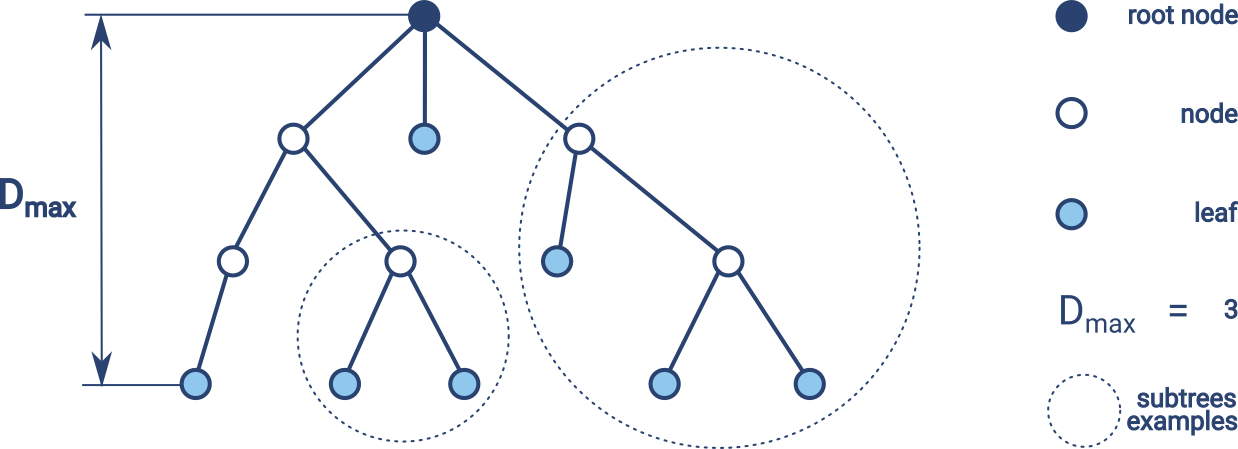Tree Walker
Simple+
English RU

This summer the 4th annual PyBay conference dedicated to Python development was held. One of the speakers was Raymond Hettinger , a member of the Python Software Foundation (PFS) since 2003, a member of the PFS board of directors from 2008 to 2012. Raymond's merits in the PFS are very significant: he worked on creating sets (data type), generator expressions, "collections" and "itertools" modules, as well as an ordered dict. He's also well known for his contributions to the Python Cookbook.

Raymond's presentation was called "The Mental Game of Python". You can watch the entire recording of the presentation here . Raymond demonstrated using various strategies to simplify the development process and make the code simpler and therefore better. This mission is a variation of a data tree traversal task used by Raymond to demonstrate an incremental development approach.

In computer science, a tree is an abstract data type that simulates a hierarchical tree structure. All tree elements are called nodes . The lines connecting elements (or nodes) are called branches and nodes without children are called leaves or end-nodes . The topmost node in a tree is called the root node . Depth is the distance between a node and the root. Additionally, every node in a tree can be seen as the root node of the subtree rooted at that node.In this mission you are given an arbitrary finite tree and a target. In case the target's type is int or str your task is to calculate how many leaves of the tree are equal to the target. Otherwise, if the target's type is list or dict you need to calculate the number of subtrees which are equal to the target.

For a better understanding, have a look at the illustrations below (visual order matters for lists). If encounter difficulties, try to watch this part of the video exposing the use of the incremental development approach.

Input: Two arguments:

• the first is an arbitrary finite tree data structure
• the second is a target , any type of [int, str, list, dict]

Output: The number (integer) of the leaves or subtrees that are equal to the given target.

Example:

```tree_walker(tree=[1, "2", 3, [, 1, {1: "one"}]], target=1) == 2                                        #example #1
tree_walker(tree={"one": 1, "two":...```
You should be an authorized user in order to see the full description and start solving this mission.